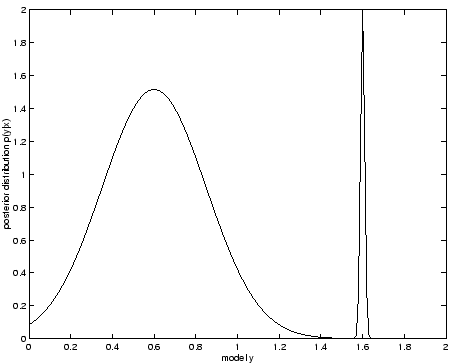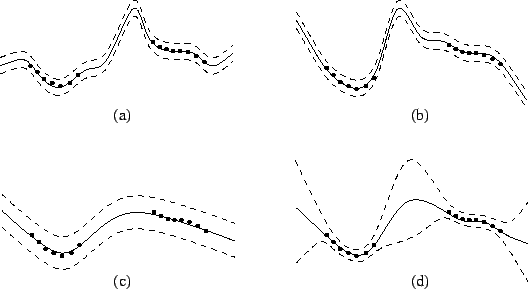Next: Approximations of posterior pdf Up: No Title Previous: Introduction

# Posterior averages in action

Probability theory tells us that the optimal generalisation is the one resulting from a Bayesian approach. Overfitting to the data means that we are making conclusions that the data does not support. Alternatively underfitting means that our conclusions are too diffuse.Overfitting is an artifact resulting from choosing only one explanation (model) for the observations. Figure 1 shows a hypothetical posterior distribution. If the model is chosen to maximise the posterior probability then the model will be chosen to be in the narrow peak. The problem is that the peak only contains a fraction of the total probability mass. This means that the model will explain the observations very well, but will be very sensitive to the values of the parameters and so may not explain further observations. When making predictions and decisions it is the position of the probability mass that counts and not the position of a maximum.

In order to solve these problems it is necessary to average over all possible hypotheses. That is we should average over all possible models, but weight them by the posterior distribution that we obtain from our observations.

It is important to note that averaging does not mean computing the average of parameter values and then using that as the best guess. For instance in digit recognition the posterior distribution may have a peak in the model for ``1''s and in the model for ``9''s. Averaging over the posterior does not mean that you compute the average digit as a ``5'' and then use that as the best guess, it means that you should prepare for the possibility that the digit can be either ``1'' or ``9''.Figure 2 shows a schematic example of fitting a Multi-Layer Perceptron (MLP) to a set of data. The data is fairly smooth and has only a little noise, but there is a region of missing data. The best regularised MLP is able to fit the data quite well in those areas where there are data points and so the noise level will be estimated to be low. Therefore the MLP will give tight error bars even in the region where there is no data to support the fit. This is overfitting because the conclusion are more specific than the data supports. If we were to later obtain some data in the missing region, it is plausible that it would lie significantly outside the MLP fit and so the posterior probability of the chosen model could rapidly drop as more data is obtained.

Instead of chosing a specific MLP it is better to average over several MLPs. In this case we would find that there are multiple explanations for the missing data. Therefore the average fit will give tight error bars where there is data and broad error bars in the regions where there is no data.

If the estimate for the level of noise in the data were estimated to be too high then the error bars for the areas with missing data might be good but the error bars for the areas with data would too broad and we would suffer from underfitting. Alternatively if the noise level is estimated to be too low, the error bars will be too narrow where there is data. For intermediate noise levels we could suffer from overfitting in some regions and underfitting in others.

In order to fix this we could allow the noise level to be part of our model and so the noise level could vary. The problems with overfitting would now be changed to overfitting the noise model. The solution is to average the noise under the posterior distribution which would include the MLP parameters and the noise.Next: Approximations of posterior pdf Up: No Title Previous: Introduction
Harri Lappalainen
2000-03-03